﻿ JavaScript Loops - TutorialsTrend
This Angular tutorial helps you get started with Angular quickly and effectively through many practical examples.
`

# JavaScript Loops

In programming, loops are control structures that allow a set of instructions to be repeated multiple times. Loops are used to iterate over a collection of data, perform a set of operations a certain number of times, or until a specific condition is satisfied.

#### Real World Examples of Loops

Suppose you run a website that sells products, and you want to display the names and prices of all the products in your catalog on a webpage. You have an array of objects called products that contains the name and price of each product. You can use a for loop in JavaScript to iterate through the array and display the name and price of each product.

Here's an example of what the loop might look like:

`````` let products = [
{ name: "Product A", price: 15 },
{ name: "Product B", price: 25.99 },
{ name: "Product C", price: 20.99 },
{ name: "Product D", price: 25.99 }
];

for (let i = 0; i < products.length; i++) {
document.write("<p> " + products[i].name + ": \$" + products[i].price + "</p>");
}``````

In this example, the for loop iterates through each element in the products array using an index variable i. For each iteration of the loop, the program displays the name and price of the product using console.log(). The output of the program would be:

Output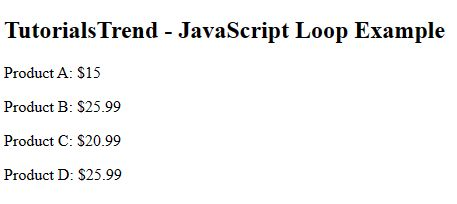#### Types of JavaScript Loops

There are five different types of loops in JavaScript.

1. While loop
2. Do-while loop
3. For loop
4. For in loop
5. For Of  loop

#### 1. The While loop

A while loop is used to repeat a set of instructions until a specific condition is met. The loop will continue to execute as long as the condition is true.

Syntax of JavaScript While Loop.

``````while(condition){
//block of code to be executed
}``````

The following flowchart describes the while loop statement.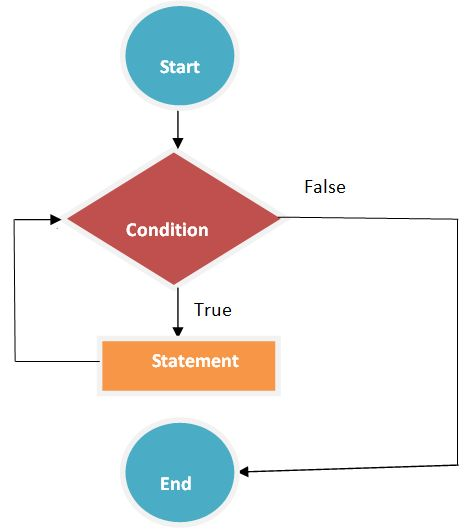Example

In this example, the while loop will continue to execute as long as count is less than 10. The value of  count starts at 0 and increments by 1 each time through the loop. The output of this program would be the numbers 0 through 9 printed to the browser.

``````<!DOCTYPE html>
<html lang="en">
<meta charset="utf-8">
<body>
<h2>TutorialsTrend - JavaScript While Loop Example</h2>
<script>
var count = 0;
while (count < 10) {
document.write("<p>The number is " + count + "</p>");
count++;
}
</script>
</body>
</html>``````

Output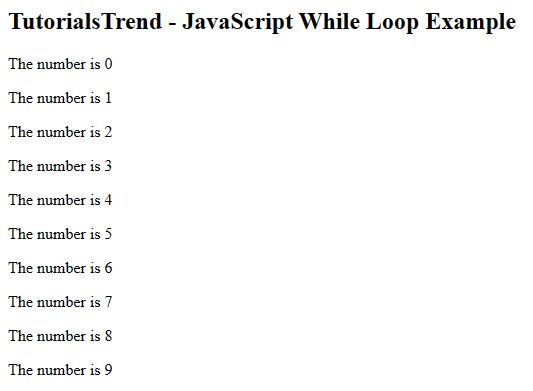#### 2. The Do-While loop

A do-while loop is similar to a while loop, but the loop will always execute at least once, regardless of whether the condition is true or false. Loops are an essential part of programming, as they allow developers to automate repetitive tasks, iterate over data, and efficiently perform complex operations.

Syntax of JavaScript Do-While Loop as following.

``````do {
// Code to be executed
}
while(condition);``````

The following flowchart describes the do-while loop statement.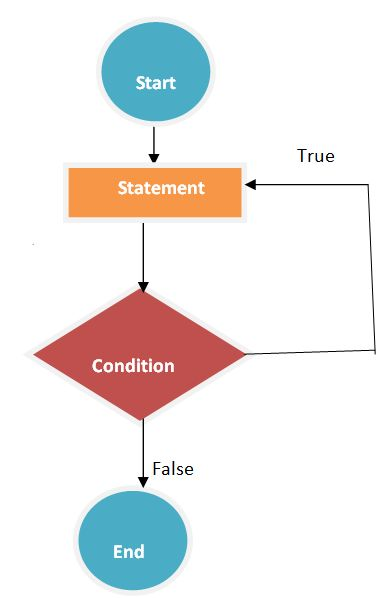Example

In this example, the do-while loop will execute at least once, printing the number 0 to the browser. The loop will continue to execute as long as count is less than 10, with the value of count incrementing by 1 each time through the loop. The output of this program would be the numbers 0 through 9 printed to the browser.

``````<!DOCTYPE html>
<html lang="en">
<meta charset="utf-8">
<body>
<h2>TutorialsTrend - JavaScript Do-While Loop Example</h2>
<script>
var count = 0;
do {
document.write("<p>The number is " + count + "</p>");
count++;
} while (count < 10)

</script>
</body>
</html>``````

Output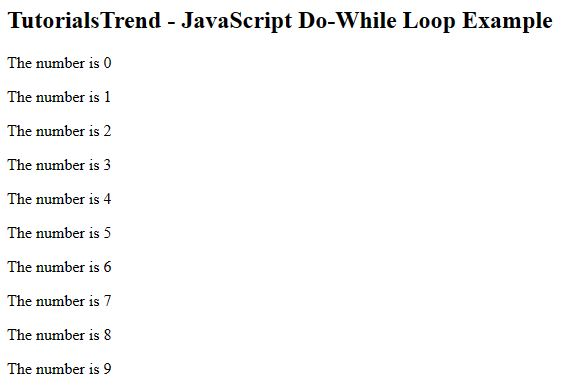#### 3. The For loop

A for loop is used to iterate a specific number of times. It is commonly used to loop over a collection of data, like an array or a list.

Syntax of JavaScript For Loop as following.

``````for(initialization; condition; increment) {
// Code to be executed
}``````

The following flowchart describes the For loop statement.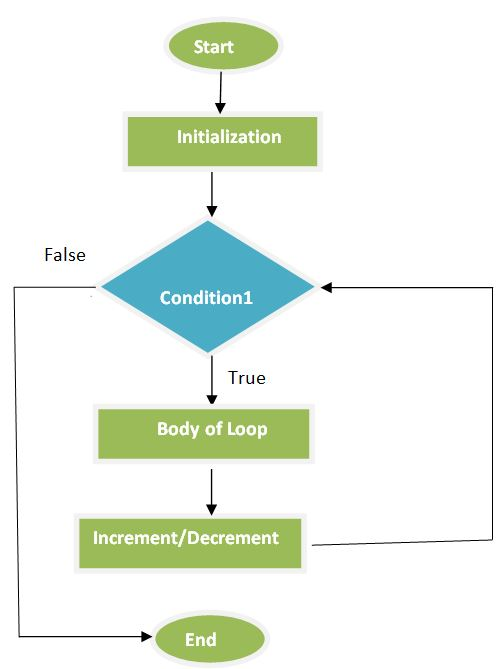Example

In this example, the for loop will execute 10 times, with the value of count starting at 0 and incrementing by 1 each time through the loop. The output of this program would be the numbers 0 through 9 printed to the browser.

``````<!DOCTYPE html>
<html lang="en">
<meta charset="utf-8">
<body>
<h2>TutorialsTrend - JavaScript For Loop Example</h2>
<script>
for (let count = 0; count < 10; count++) {
document.write("<p>The number is " + count + "</p>");
}
</script>
</body>
</html>``````

Output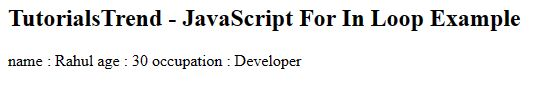#### 4. The For In loop

The for...in loop in JavaScript is used to iterate over the properties of an object.

Here's the basic syntax:

``````for (variable in object) {
// code to be executed
}``````

In this syntax, variable is a variable that will be assigned to each property name in object during each iteration of the loop. The object parameter is the object you want to iterate over.

Here's an example of how you might use a for...in loop to iterate over the properties of an object:

Example

In this example, the loop will iterate over the properties of the person object (name, age, and occupation) and log each property name and its corresponding value to the browser window.

``````<!DOCTYPE html>
<html lang="en">
<meta charset="utf-8">
<body>
<h2>TutorialsTrend - JavaScript For In Loop Example</h2>
<script>
let person = {
name: 'Rahul',
age: 30,
occupation: 'Developer'
};

for (let property in person) {
document.write(`\${property} : \${person[property]} `);
}
</script>
</body>
</html>``````

Output#### 5. The For Of loop

The for...of loop in JavaScript is used to iterate over iterable objects such as arrays, strings, maps, sets, etc.

Note - the for...of loop cannot be used to iterate over the properties of an object. For that, you would need to use a for...in loop

Here's the basic syntax:

``````for (variable of iterable) {
// code to be executed
}``````

In this syntax, variable is a variable that will be assigned to each element of iterable during each iteration of the loop. The iterable parameter is the iterable object you want to iterate over.

Here's an example of how you might use a for...of loop to iterate over the elements of an array:

Example

In this example, the loop will iterate over the elements of the numbers array (1, 2, 3, 4, and 5) and log each element to the browser window.

``````<!DOCTYPE html>
<html lang="en">
<meta charset="utf-8">
<body>
<h2>TutorialsTrend - JavaScript For For Loop Example</h2>
<script>
let numbers = [1, 2, 3, 4, 5];

for (let number of numbers) {
document.write(number +"</br>");
}
</script>
</body>
</html>``````

Output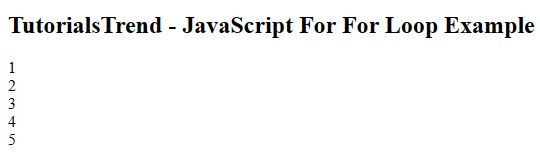Prev Next